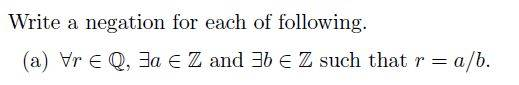# Negation for propositionmy attempt.

Let P = At least one a and at least one b
Let Q = r=a/b

Hence the proposition is simplified to,

For all r where P Then Q

Negation:
Not all
r where P Then Q
= Atleast one R When Not(P Then Q)

Not(P Then Q) = P And Not Q

Hence
Atleast one R When Not(P Then Q)
= Atleast one R When P And Not Q

After this step i substitute back P and Q.
Is this way correct?

WWGD
Gold Member
There is a general method for negating that goes from the outermost (left-most) statement : For all is negated into there ismy attempt.

Let P = At least one a and at least one b
Let Q = r=a/b

Hence the proposition is simplified to,

For all r where P Then Q

Negation:
Not all
r where P Then Q
= Atleast one R When Not(P Then Q)

Not(P Then Q) = P And Not Q

Hence
Atleast one R When Not(P Then Q)
= Atleast one R When P And Not Q

After this step i substitute back P and Q.
Is this way correct?

There is a general method for negating compound statements : the negation is done from the leftmost to rightmost statement: first you negate the "for all" into there exists
and then you negate the A and B statement into NotA or NotB , where A is There exists an integer Z , negated into " For all a in Z "and so on. Informally, the negation says that there exists a Rational that is not the ratio of two integers.

Hi so the ans is.

There exists a rational number r, Where all integer a and all integer b such that r != a/b

May i know what is "such that' converted to? For my case i tot it means "then"